# JP2010060489A - Satellite orbital modeling propriety determination method, long term prediction orbital data supply method, and satellite orbital modeling propriety determination device - Google Patents

Satellite orbital modeling propriety determination method, long term prediction orbital data supply method, and satellite orbital modeling propriety determination device Download PDF

## Info

Publication number
JP2010060489A
JP2010060489A JP2008228006A JP2008228006A JP2010060489A JP 2010060489 A JP2010060489 A JP 2010060489A JP 2008228006 A JP2008228006 A JP 2008228006A JP 2008228006 A JP2008228006 A JP 2008228006A JP 2010060489 A JP2010060489 A JP 2010060489A
Authority
JP
Japan
Prior art keywords
predicted
modeling
position
satellite
orbit
Prior art date
Legal status (The legal status is an assumption and is not a legal conclusion. Google has not performed a legal analysis and makes no representation as to the accuracy of the status listed.)
Pending
Application number
JP2008228006A
Other languages
Japanese (ja)
Inventor
Kenji Onda

Original Assignee
Seiko Epson Corp
セイコーエプソン株式会社
Priority date (The priority date is an assumption and is not a legal conclusion. Google has not performed a legal analysis and makes no representation as to the accuracy of the date listed.)
Filing date
Publication date
Application filed by Seiko Epson Corp, セイコーエプソン株式会社 filed Critical Seiko Epson Corp
Priority to JP2008228006A priority Critical patent/JP2010060489A/en
Publication of JP2010060489A publication Critical patent/JP2010060489A/en
Pending legal-status Critical Current

• 238000000034 methods Methods 0 description 60
• 238000006243 chemical reaction Methods 0 description 1
• 230000000694 effects Effects 0 description 1

## Images

•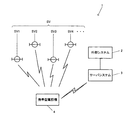•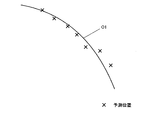•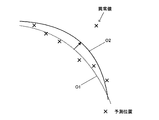••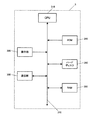•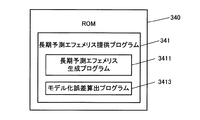••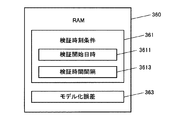•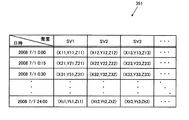•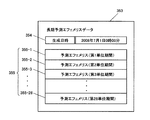••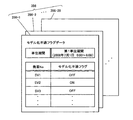••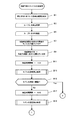•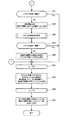•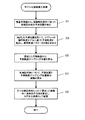•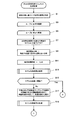•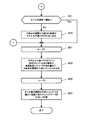•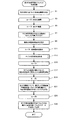•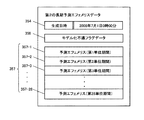## Classifications

• GPHYSICS
• G01MEASURING; TESTING
• G01S19/00Satellite radio beacon positioning systems; Determining position, velocity or attitude using signals transmitted by such systems
• G01S19/01Satellite radio beacon positioning systems transmitting time-stamped messages, e.g. GPS [Global Positioning System], GLONASS [Global Orbiting Navigation Satellite System] or GALILEO
• G01S19/03Cooperating elements; Interaction or communication between different cooperating elements or between cooperating elements and receivers
• G01S19/08Cooperating elements; Interaction or communication between different cooperating elements or between cooperating elements and receivers providing integrity information, e.g. health of satellites or quality of ephemeris data
• GPHYSICS
• G01MEASURING; TESTING
• G01S19/00Satellite radio beacon positioning systems; Determining position, velocity or attitude using signals transmitted by such systems
• G01S19/01Satellite radio beacon positioning systems transmitting time-stamped messages, e.g. GPS [Global Positioning System], GLONASS [Global Orbiting Navigation Satellite System] or GALILEO
• G01S19/02Details of the space or ground control segments
• GPHYSICS
• G01MEASURING; TESTING
• G01S19/00Satellite radio beacon positioning systems; Determining position, velocity or attitude using signals transmitted by such systems
• G01S19/01Satellite radio beacon positioning systems transmitting time-stamped messages, e.g. GPS [Global Positioning System], GLONASS [Global Orbiting Navigation Satellite System] or GALILEO
• G01S19/03Cooperating elements; Interaction or communication between different cooperating elements or between cooperating elements and receivers
• G01S19/05Cooperating elements; Interaction or communication between different cooperating elements or between cooperating elements and receivers providing aiding information
• GPHYSICS
• G01MEASURING; TESTING
• G01S19/00Satellite radio beacon positioning systems; Determining position, velocity or attitude using signals transmitted by such systems
• G01S19/01Satellite radio beacon positioning systems transmitting time-stamped messages, e.g. GPS [Global Positioning System], GLONASS [Global Orbiting Navigation Satellite System] or GALILEO
• G01S19/24Acquisition or tracking or demodulation of signals transmitted by the system
• GPHYSICS
• G01MEASURING; TESTING
• G01S19/00Satellite radio beacon positioning systems; Determining position, velocity or attitude using signals transmitted by such systems
• G01S19/01Satellite radio beacon positioning systems transmitting time-stamped messages, e.g. GPS [Global Positioning System], GLONASS [Global Orbiting Navigation Satellite System] or GALILEO
• G01S19/24Acquisition or tracking or demodulation of signals transmitted by the system
• G01S19/25Acquisition or tracking or demodulation of signals transmitted by the system involving aiding data received from a cooperating element, e.g. assisted GPS
• G01S19/258Acquisition or tracking or demodulation of signals transmitted by the system involving aiding data received from a cooperating element, e.g. assisted GPS relating to the satellite constellation, e.g. almanac, ephemeris data, lists of satellites in view
• GPHYSICS
• G01MEASURING; TESTING
• G01S19/00Satellite radio beacon positioning systems; Determining position, velocity or attitude using signals transmitted by such systems
• G01S19/01Satellite radio beacon positioning systems transmitting time-stamped messages, e.g. GPS [Global Positioning System], GLONASS [Global Orbiting Navigation Satellite System] or GALILEO
• G01S19/24Acquisition or tracking or demodulation of signals transmitted by the system
• G01S19/27Acquisition or tracking or demodulation of signals transmitted by the system creating, predicting or correcting ephemeris or almanac data within the receiver

## Abstract

A method for determining the suitability of satellite orbit modeling is proposed.
In a positioning system 1, a server system 3 approximates a satellite orbit of a GPS satellite SV based on a satellite prediction calendar including a predicted position of the GPS satellite SV acquired from an external system 2 in time series. The predicted orbit is obtained according to the approximate model, and the value of the satellite orbit parameter is calculated. Then, a predicted orbit sampling position (calculated position) that is a future position of the GPS satellite SV is calculated using the calculated satellite orbit parameter value, and the satellite orbit is calculated based on the distance between the predicted position and the predicted orbit sampling position. Determine the suitability of modeling.
[Selection] Figure 1

## Description

The present invention relates to a satellite orbit modeling suitability determination method, a long-term predicted orbit data providing method, and a satellite orbit modeling suitability determination apparatus.

As a positioning system using positioning signals, GPS (Global Positioning System) is widely known, and is used in positioning devices built in portable telephones, car navigation devices, and the like. In GPS, the values of four parameters, the three-dimensional coordinate value indicating the position of the aircraft and the clock error, are calculated based on information such as the positions of a plurality of GPS satellites and the pseudoranges from each GPS satellite to the aircraft. Positioning is performed by performing the required positioning calculation.

In positioning by GPS, satellite information such as the position, velocity, and moving direction of the GPS satellite is calculated based on navigation data such as almanac and ephemeris superimposed on the GPS satellite signal transmitted from the GPS satellite. Positioning calculation is performed using time information. In particular, the ephemeris is a powerful clue when capturing a satellite. For example, when positioning is started without holding the ephemeris, the first positioning time (TTFF: Time To First Fix) increases. Even when the positioning is not the first time, when acquiring a satellite for the first time, the acquisition time varies greatly depending on whether or not the ephemeris of the satellite is held.

Therefore, Patent Document 1 refers to a server client system in which a server predicts a long-term ephemeris such as one week, and this predicted ephemeris (hereinafter referred to as “long-term predicted ephemeris” (long-term predicted orbit data)). ) Is provided to a positioning device that is a client.
International Publication No. 2006/031652 Pamphlet

As a method of defining the long-term predicted ephemeris, a method of defining in a data format similar to that of a normal ephemeris can be considered. That is, a satellite orbit is approximated using Kepler's elliptical orbit model, which is one of the approximate models of the satellite orbit, and long-term prediction is performed based on the value of the model equation parameter (hereinafter referred to as “satellite orbit parameter”). It is a method of defining an ephemeris. A satellite prediction calendar (predicted position data) including predicted positions obtained by predicting a future position of a positioning satellite in time series every predetermined time is provided from a predetermined commercial system. Approximate calculations using Kepler's elliptical orbit model can be performed using this satellite prediction calendar.

However, among the predicted positions stored in the satellite predicted calendar, predicted positions that are extremely distant, such as several kilometers, may be included as “abnormal values” because of the positional relationship with adjacent predicted positions. An ideal satellite orbit approximation is an elliptical orbit that accurately reproduces all predicted positions. However, when an abnormal value is included, the elliptical shape is distorted by being dragged to the abnormal value. Obviously, the distorted satellite orbit has poor reproducibility of the predicted position.

The present invention has been made in view of the above-described problems, and its purpose is to propose a method for determining the suitability of satellite orbit modeling that approximates the future orbit of a positioning satellite. is there.

A first invention for solving the above problems is a predetermined model used when approximating the satellite orbit of the positioning satellite based on the predicted position data including the predicted position of the positioning satellite in time series. Calculating the parameter value of the equation; calculating the calculated position of the positioning satellite derived from the model equation using the parameter value; and the difference between the predicted position and the calculated position. And determining whether or not the satellite orbit modeling is appropriate based on the model formula using the parameter value.

As another invention, based on predicted position data including the predicted position of the positioning satellite in time series, the value of a parameter included in a predetermined model formula used when approximating the satellite orbit of the positioning satellite is calculated. Based on the difference between the predicted position and the calculated position, the calculated parameter value calculating section, the calculated position calculating section for calculating the calculated position of the positioning satellite derived from the model formula using the parameter value, In addition, a satellite orbit modeling suitability determining device may be configured to include a determination unit that determines whether or not the satellite orbit modeling is appropriate based on the model formula using the parameter value.

According to the first aspect of the present invention, based on the predicted position data of the positioning satellite, the parameter value of the predetermined model formula used when approximating the satellite orbit of the positioning satellite is calculated. Then, the calculated position of the positioning satellite derived from the model equation is calculated using the calculated parameter value, and based on the difference between the predicted position and the calculated position, the satellite orbit by the model equation using the parameter value is calculated. Determine the suitability of modeling.

If the satellite orbit modeling is appropriate, the difference between the predicted position of the positioning satellite included in the predicted position data and the calculated position of the positioning satellite derived from the model formula should be small. Therefore, it is possible to determine the suitability of satellite orbit modeling by using the difference between the predicted position and the calculated position as a criterion.

As a second invention, in the satellite orbit modeling suitability determination method according to the first invention, the model formula is a function for inputting a time and outputting a position, and calculating the calculated position is Calculating the calculated position at the same time as the predicted position, and determining whether the modeling is appropriate is determining whether the modeling is appropriate based on a distance between the predicted position and the calculated position. A satellite orbit modeling suitability determination method may be configured.

According to the second aspect of the invention, the calculated position at the same time as the predicted position is calculated, and the suitability of the satellite orbit modeling is determined based on the distance between the predicted position and the calculated position. If satellite orbit modeling is appropriate, the distance between the predicted position and the calculated position of the positioning satellite at the same time should be small. Therefore, for example, by performing threshold determination with respect to the sum of distances calculated for each time, it is possible to realize effective suitability determination for satellite orbit modeling.

The third aspect of the invention is the satellite orbit modeling suitability determination method according to the second aspect of the invention, wherein among the predicted positions included in the predicted position data, within a predetermined distance from the calculated position at the same time as the predicted position. A satellite orbit model including: selecting a predicted position; and calculating the value of the parameter, calculating the calculated position, and determining whether the modeling is appropriate based on the selected predicted position You may comprise the optimization suitability determination method.

According to the third aspect, a predicted position within a predetermined distance from the calculated position at the same time as the predicted position is selected. Then, based on the selected predicted position, the calculation of the parameter value, the calculation position, and the modeling suitability determination are performed again. When the predicted position and the calculated position of the positioning satellite at the same time are far apart, there is a high possibility that the predicted position is an abnormal value greatly deviating from the ideal satellite orbit. Therefore, it is preferable to exclude such abnormal values and perform satellite orbit modeling again.

According to a fourth invention, there is provided a satellite orbit modeling suitability determination method according to any one of the first to third inventions, wherein a predicted position used for calculation of the parameter value is calculated from the predicted position data. Satellite orbit modeling including: determining a combination; and calculating the value of the parameter, calculating the calculated position, and determining whether the modeling is appropriate based on the determined combination of predicted positions A suitability determination method may be configured.

According to the fourth aspect of the invention, the combination of predicted positions used for calculating the parameter value is determined. Then, based on the determined combination of predicted positions, calculation of parameter values, calculation position calculation, and modeling suitability determination are performed.

According to a fifth aspect of the present invention, there is provided a satellite orbit modeling suitability determination method according to the fourth aspect of the present invention, wherein when the modeling is determined to be inappropriate, a combination of predicted positions used for calculating the parameter value And changing the parameter value, calculating the calculated position, and re-determining whether or not the modeling is appropriate based on the combination of the changed predicted positions. A suitability determination method may be configured.

According to the fifth aspect of the invention, when it is determined that modeling is not appropriate, the combination of predicted positions used for calculating the parameter value is changed. Then, based on the changed combination of predicted positions, the calculation of parameter values, the calculation position, and the suitability for modeling are performed again. As described above, when it is determined that the satellite orbit modeling is not appropriate, an appropriate parameter value can be obtained by changing the combination of the predicted positions and performing the modeling again.

Further, as a sixth invention, the satellite orbit modeling suitability determination method according to any one of the first to fourth inventions is used to calculate the parameter value, the calculation position, and the calculation for each successive predetermined unit period. Long-term predicted trajectory data including: determining whether the modeling is appropriate; and providing long-term predicted trajectory data that summarizes the values of the parameters of the unit period determined to be appropriate in the modeling appropriateness determination. A providing method may be configured.

According to the sixth aspect of the invention, the parameter value calculation, the calculation position calculation, and the modeling suitability determination are performed for each of the predetermined continuous unit periods. Then, long-term predicted trajectory data is provided that summarizes the values of the parameters of each unit period determined to be appropriate in the modeling appropriateness determination. As a result, it is possible to provide only the value of the parameter of the unit period that has been appropriately modeled to the positioning device.

Further, as a seventh invention, by the satellite orbit modeling suitability determination method according to any one of the first to fourth inventions, the calculation of the parameter value, the calculation position, and the calculation for a predetermined continuous unit period, and Performing the modeling suitability determination, and providing long-term predicted trajectory data in which the parameter values of the predetermined unit period are associated with the modeling suitability determination result for the unit period. , Long-term predicted orbit data providing method may be configured.

According to the seventh aspect of the invention, the parameter value calculation, the calculation position calculation, and the modeling suitability determination are performed for each successive predetermined unit period. Then, long-term predicted trajectory data in which the parameter values of a predetermined unit period are associated with the modeling determination result for the unit period is provided. By providing the parameter values of a plurality of unit periods in association with the modeling suitability determination result for the unit period, the positioning device can determine the unit period in which the modeling is appropriately performed. It becomes. And about the unit period in which modeling was not performed appropriately, the value of the parameter of the unit period can be prevented from being used for positioning.

Hereinafter, an example of an embodiment suitable for the present invention will be described with reference to the drawings. However, embodiments to which the present invention can be applied are not limited to this.

1. System Configuration FIG. 1 is a diagram showing a schematic configuration of a positioning system 1 in the present embodiment. The positioning system 1 includes an external system 2, a server system 3, a mobile phone 4 that is an electronic device equipped with a positioning device, and a plurality of GPS satellites SV (SV1, SV2, SV3, SV4,...). It is prepared for. In addition, since the mobile phone 4 and the GPS satellite SV can perform positioning after the data necessary for the mobile phone 4 is acquired from the server system 2, one positioning is performed by the mobile phone 4 and the GPS satellite SV. It can also be said that the system is configured. Further, as a system on the ground side, the server system 3 and the mobile phone 4 can be called a positioning system.

The external system 2 is a known system that periodically receives a satellite signal from the GPS satellite SV, generates a satellite prediction calendar based on navigation data included in the satellite signal, and provides it to the server system 3. The satellite prediction calendar provided by the external system 2 is data of discontinuous positions in which predicted positions for which future positions are predicted are arranged in time series every predetermined time for each GPS satellite SV. The external system 2 corresponds to, for example, a computer system of a private or public organization that provides the satellite forecast calendar.

The server system 3 acquires the satellite prediction calendar from the external system 2, and uses the satellite prediction calendar to predict the ephemeris of all the GPS satellites SV, which is a long period of at least one day, for example, one week. The system includes a server that generates and provides an effective ephemeris (hereinafter referred to as “long-term predicted ephemeris (long-term predicted orbit data)” in the present embodiment).

The mobile phone 4 is an electronic device for a user to send and receive calls and mails. The mobile phone 4 has a function for measuring a position (positioning function) in addition to the original functions as a mobile phone such as sending and receiving calls and emails. It has a positioning device to realize. The mobile phone 4 transmits a long-term predicted ephemeris request signal to the server system 3 and receives the long-term predicted ephemeris from the server system 3 in accordance with a user operation. Then, the GPS satellite SV is captured using the received long-term predicted ephemeris, and positioning calculation based on the satellite signal is executed.

2. Principle FIG. 2 and FIG. 3 are diagrams showing an example of a satellite prediction calendar generated by the external system 2 and a satellite orbit calculated based on the satellite prediction calendar. FIG. 2 shows a case where an abnormal value of a predicted position is not included in the satellite prediction calendar, and FIG. 3 shows an example where an abnormal value is included. In the present embodiment, among the predicted positions included in the satellite prediction calendar, a predicted position that is extremely far away from the adjacent predicted position is referred to as an “abnormal value”. The distance between a predicted position that is not an abnormal value and a predicted trajectory sampling position described later is about several meters to several tens of meters, but the distance between the predicted value of an abnormal value and the predicted trajectory sampling position is several. km.

In the present embodiment, Kepler's satellite orbit model formula (hereinafter also referred to as “approximate model”) that minimizes the sum of squares of the distances from all predicted positions stored in the satellite prediction calendar is referred to as a minimum two. Obtained using multiplication. The parameters of the approximate model equation of the satellite orbit obtained at this time are referred to as satellite orbit parameters, and the calculation for calculating the approximate model is also referred to as “approximate calculation”. The satellite orbit obtained by the approximate calculation is referred to as “predicted orbit”.

The approximate model used in the present embodiment is a model representing an elliptical orbit and does not necessarily pass through the predicted position included in the satellite orbit calendar. The predicted orbit is represented by a function having time as a variable, and the position of the GPS satellite SV is returned as an output value by giving the time as an input value.

When an abnormal value is not included in the predicted position included in the satellite prediction calendar, for example, a predicted orbit O1 that is an average orbit for all predicted positions as shown in FIG. 2 is obtained. On the other hand, when an abnormal value is included in the predicted position stored in the satellite prediction calendar, for example, a distorted elliptical shape in which a part of the predicted trajectory swells in the direction of the abnormal value as shown in FIG. Of the predicted trajectory O2.

FIG. 4 is a diagram for explaining the principle of determining the propriety of modeling the predicted trajectory. In the present embodiment, a satellite orbit modeling error based on the difference between the predicted position included in the satellite predicted calendar and the predicted orbit sampling position (calculated position) calculated from the predicted orbit obtained by the approximate calculation. (Hereinafter referred to as “modeling error”). The predicted position and the predicted orbit sampling position are positions of GPS satellites (presumed positions) at the same time. For example, every 15 minutes every hour. A modeling error is calculated based on the difference between the predicted position and the predicted orbit sampling position at each same time, and it is determined whether or not the approximated satellite orbit is appropriate. More precisely, it is determined whether or not the parameter value of the approximate model formula is appropriate.

More specifically, in the satellite orbital calendar, predicted positions at predetermined time intervals (for example, “every 15 minutes”) are stored in time series. In FIG. 4, the predicted positions “P t1 to P t7 ” at each time from time “ t1 to t7 ” are indicated by “x”. The predicted trajectory sampling positions “S t1 to St 7” are obtained by calculating points at the same time “ t1 to t7 ” as the predicted position from the predicted trajectory obtained by the approximate calculation. FIG. 4 shows the predicted orbit sampling position "S t1 to S t7" in black circle.

Next, for each of the times “t1 to t7”, distances “r 1 to r 7 ” between the predicted position and the predicted trajectory sampling position are calculated. The modeling error is expressed as, for example, the square root of the sum of squares of all the calculated distances. Specifically, when there are “n” times (sampling points) in total, a modeling error is calculated according to the following equation (1).

If the modeling error is large, it is considered that the predicted trajectory is deviated from the predicted position as a whole, or there is a part in the predicted trajectory that is largely deviated from the predicted position. Judgment can be made. On the other hand, when the modeling error is small, it indicates that the predicted trajectory is a trajectory that is close to the entire predicted position or passes through the same position, and the predicted trajectory appropriately reproduces the predicted position. Judgment can be made.

Therefore, threshold determination is performed for the modeling error, and when the modeling error does not reach a predetermined threshold (for example, “2000 [m]”), satellite orbit modeling is appropriate (prediction). It is determined that the position reproducibility is good. On the other hand, when the modeling error has reached a predetermined threshold, it is determined that the modeling of the satellite orbit is inappropriate (the reproducibility of the predicted position is poor).

3. Functional Configuration FIG. 5 is a block diagram showing a functional configuration of the server system 3. The server system 3 includes a CPU (Central Processing Unit) 310, an operation unit 320, a communication unit 330, a ROM (Read Only Memory) 340, a hard disk 350, and a RAM (Random Access Memory) 360. A computer system connected by a bus 370.

The CPU 310 is a processor that comprehensively controls each unit of the server system 3 according to a system program or the like stored in the ROM 340. In the present embodiment, the CPU 310 performs a process of providing the long-term predicted ephemeris to the mobile phone 4 according to the long-term predicted ephemeris providing program 341 stored in the ROM 340.

The operation unit 320 is an input device that receives an operation instruction from an administrator of the server system 3 and outputs a signal corresponding to the operation to the CPU 310. This function is realized by, for example, a keyboard, a button, a mouse, or the like.

The communication unit 330 is a communication device for exchanging various data used in the system with the external system 2 and the mobile phone 4 via a communication network such as the Internet.

The ROM 340 is a read-only nonvolatile storage device, and generates a system program for the CPU 310 to control the server system 3, a program for providing the long-term predicted ephemeris to the mobile phone 4, and a long-term predicted ephemeris. And various programs such as a program for calculating modeling errors, data, and the like are stored.

The hard disk 350 is a storage device that reads and writes data using a magnetic head or the like, and stores programs, data, and the like for realizing various functions of the server system 3, similar to the ROM 340.

The RAM 360 is a readable / writable volatile storage device, and a work area for temporarily storing a system program executed by the CPU 310, a long-term prediction ephemeris providing program, various processing programs, data being processed in various processing, processing results, and the like. Is forming.

4). Data Configuration FIG. 6 is a diagram illustrating an example of data stored in the ROM 340. The ROM 340 stores a long-term predicted ephemeris providing program 341 read by the CPU 310 and executed as a long-term predicted ephemeris providing process (see FIG. 13). The long-term predicted ephemeris providing program 341 is executed as a long-term predicted ephemeris generating program 3411 executed as a long-term predicted ephemeris generating process (see FIGS. 14 and 15) and a modeling error calculating process (see FIG. 16). A modeling error calculation program 3413 is included as a subroutine.

The long-term predicted ephemeris providing process is generated when the CPU 310 periodically performs the process of generating the long-term predicted ephemeris data 353 and receives a request signal for the long-term predicted ephemeris data 353 from the mobile phone 4. This is processing for transmitting the long-term predicted ephemeris data 353 to the requesting mobile phone 4.

The long-term predicted ephemeris generation process is a process in which the CPU 310 generates long-term predicted ephemeris data 353. In the present embodiment, the CPU 310 generates long-term predicted ephemeris data 353 once every 4 hours. Specifically, 28 weeks of predicted ephemeris 355 are generated by setting the period until one week later as the prediction period based on the generation date and time of the long-term predicted ephemeris data 353, and dividing the prediction period into 6 hours as unit periods. To do. Then, long-term predicted ephemeris data 353 in which the predicted ephemeris 355 for 28 unit periods is collected is generated.

The modeling error calculation process is a process in which the CPU 310 calculates a satellite orbit modeling error in accordance with the principle described above. These processes will be described later in detail using a flowchart.

FIG. 7 is a diagram illustrating an example of data stored in the hard disk 350. The hard disk 350 stores satellite prediction calendar 351, long-term prediction ephemeris data 353, and modeling inappropriate flag data 356.

FIG. 9 is a diagram illustrating an example of a data configuration of the satellite prediction calendar 351. The satellite prediction calendar 351 is discrete data in which the predicted positions of each GPS satellite SV up to one week later are stored every 15 minutes. The predicted position is represented by, for example, a three-dimensional coordinate value in the earth reference coordinate system. For example, the predicted position of the GPS satellite “SV2” at “0:30 on July 1, 2008” is “(X32, Y32, Z32)”. The CPU 310 receives the satellite forecast calendar 351 from the external system 2 periodically (for example, once every four hours), and stores / updates it in the hard disk 350.

FIG. 10 is a diagram illustrating an example of the data configuration of the long-term predicted ephemeris data 353. In the long-term predicted ephemeris data 353, the generation date 354 of the long-term predicted ephemeris data 353 and the predicted ephemeris 355 (355-1 to 355-28) of the first to 28th unit periods are stored in association with each other.

FIG. 11 is a diagram illustrating an example of the data configuration of the predicted ephemeris 355. The predicted ephemeris 355 (355-1, 355-2,..., 355-28) has Kepler satellite orbit parameter values such as orbit length radius, eccentricity, and orbit inclination angle for each of the satellites SV1 to SV32. It is remembered.

However, for each predicted ephemeris of each unit period, the satellite orbit parameter value is not stored for the GPS satellite SV determined to be inappropriate for satellite orbit modeling. For example, in the predicted ephemeris 355-1 in the first unit period, since it is determined that the satellite orbit modeling of the GPS satellite SV2 is inappropriate, the value of the satellite orbit parameter is not stored for the GPS satellite SV2.

FIG. 12 is a diagram illustrating an example of a data configuration of the modeling inappropriate flag data 356. As illustrated in FIG. In the modeling inappropriate flag data 356 (356-1, 356-2,..., 356-28), the number of the GPS satellite SV and the modeling inappropriate flag are stored in association with each unit period. Yes.

The improper modeling flag is “ON” for GPS satellites SV determined to be inappropriate for satellite orbit modeling, and “OFF” for GPS satellites SV determined to be appropriate for satellite orbit modeling. The flag to be set. For example, in the modeling inappropriate flag data 356-1 for the first unit period of FIG. 12, since it is determined that the satellite orbit modeling for the GPS satellite SV2 is inappropriate, the modeling inappropriate flag for the GPS satellite SV2 is “ON”. "

FIG. 8 is a diagram illustrating an example of data stored in the RAM 360. The RAM 360 stores a verification time condition 361 and a modeling error 363.

The verification time condition 361 is a condition related to the time for verifying the suitability of modeling of the satellite orbit, and the verification start date and time 3611 that is the date and time when the verification is started among the dates and times stored in the satellite prediction calendar 351 and the verification. A verification time interval 3613 that is a time interval to be performed is stored. The verification time condition 361 is variably set by the CPU 310 in the long-term predicted ephemeris generation process.

The modeling error 363 is a satellite orbit modeling error, and is calculated and stored as, for example, the square root of the sum of squares of the distance between the predicted position calculated for each verification time and the predicted orbit sampling position.

5). Process Flow FIG. 13 is a flowchart showing the flow of the long-term predicted ephemeris providing process executed in the server system 3 by the CPU 310 reading and executing the long-term predicted ephemeris providing program 341 stored in the ROM 340. is there.

First, the CPU 310 determines whether or not the satellite prediction calendar 351 has been received from the external system 2 (step A1). If it is determined that the satellite prediction calendar 351 has not been received (step A1; No), the process proceeds to step A5. . If it is determined that it has been received (step A1; Yes), the received satellite prediction calendar 351 is updated and stored in the hard disk 350 (step A3).

Next, the CPU 310 determines whether or not it is the generation time of the long-term predicted ephemeris (step A5). In the present embodiment, the long-term predicted ephemeris is generated once every 4 hours. If it is determined that the generation time is not yet reached (step A5; No), the CPU 310 proceeds to step A9.

When it is determined that it is the generation time of the long-term predicted ephemeris (step A5; Yes), the CPU 310 reads out and executes the long-term predicted ephemeris generation program 3411 stored in the ROM 340, thereby executing the long-term predicted ephemeris generation process. (Step A7).

14 and 15 are flowcharts showing the flow of long-term predicted ephemeris generation processing.
First, the CPU 310 determines each unit period based on the current date and time (step B1). Specifically, a period from the current date and time (generation date and time) to one week later is set as a prediction period, and each period obtained by dividing the prediction period every 6 hours is determined as a unit period.

Next, the CPU 310 executes the process of loop A for each unit period determined in step B1 (steps B3 to B35). In the process of loop A, the CPU 310 executes the process of loop B for each GPS satellite SV (steps B5 to B31).

In the process of loop B, the CPU 310 sets the modeling inappropriate flag of the GPS satellite SV of the unit period to “OFF” in the modeling inappropriate flag data 356 of the hard disk 350 (step B7). Then, the CPU 310 stores the oldest date / time included in the satellite prediction calendar 351 stored in the hard disk 350 as the verification start date / time 3611 and stores it in the RAM 360 as the verification time condition 361 (step B9).

Further, the CPU 310 sets the verification time interval 3613 to “15 minutes” and stores it in the RAM 360 as the verification time condition 361 (step B11). Then, the CPU 310 reads out and executes the modeling error calculation program 3413 stored in the ROM 340, thereby performing a modeling error calculation process (step B13).

FIG. 16 is a flowchart showing the flow of the modeling error calculation process.
First, the CPU 310 extracts a predicted position at each verification time according to the verification time condition 361 stored in the RAM 360 from the satellite predicted calendar 351 stored in the hard disk 350 (step C1).

Next, the CPU 310 calculates a predicted orbit based on the Kepler elliptical orbit model using the predicted position extracted in step C1, and obtains the value of the satellite orbit parameter (step C3). Then, the CPU 310 calculates a predicted orbit sampling position at the same time as the verification time from the predicted orbit calculated in step C3 (step C5).

Then, the CPU 310 calculates the distance between the predicted position and the predicted trajectory sampling position for each verification time (step C7). Then, the CPU 310 calculates the square root of the square sum of the distances calculated for all the verification times, and stores it in the RAM 360 as the modeling error 363 (step C9). Then, the CPU 310 ends the modeling error calculation process.

Returning to the long-term predicted ephemeris generation process of FIG. 14, after performing the modeling error calculation process, the CPU 310 determines whether or not the modeling error 363 stored in the RAM 360 is less than a predetermined threshold (step). B15) If it is determined that it is less than the threshold value (step B15; Yes), the process proceeds to the next GPS satellite SV.

If it is determined that the modeling error 363 is greater than or equal to the threshold (step B15; No), the CPU 310 updates the verification time interval 3613 stored in the RAM 360 to “30 minutes” (step B17). That is, the verification time condition is set so that the predicted trajectory is calculated using a combination of predicted positions extracted at 30-minute intervals instead of using all predicted positions stored in the satellite prediction calendar 351.

Then, the CPU 310 reads out and executes the modeling error calculation program 3413 stored in the ROM 340, thereby performing a modeling error calculation process (step B19). Thereafter, the CPU 310 determines whether or not the modeling error 363 stored in the RAM 360 is less than a predetermined threshold (step B21). If it is determined that the modeling error 363 is less than the threshold (step B21; Yes), the next The process is shifted to the GPS satellite SV.

If it is determined that the modeling error 363 is greater than or equal to the threshold (step B21; No), the CPU 310 sets the verification start date and time 3611 stored in the RAM 360 as “the second oldest date and time included in the satellite prediction calendar”. (Step B23). That is, when extracting the predicted position included in the satellite prediction calendar 351 at 30-minute intervals, the prediction time condition is set so that it is extracted not from the oldest time but from the second oldest time. Change the combination of predicted positions used to calculate the trajectory.

Then, the CPU 310 reads out and executes the modeling error calculation program 3413 stored in the ROM 340, thereby performing modeling error calculation processing (step B25). Thereafter, the CPU 310 determines whether or not the modeling error 363 stored in the RAM 360 is less than a predetermined threshold (step B27). If it is determined that the modeling error 363 is less than the threshold (step B27; Yes), the next The process is shifted to the GPS satellite SV.

If it is determined that the modeling error 363 is greater than or equal to the threshold (step B27; No), the CPU 310 models the GPS satellite SV of the unit period stored in the modeling inappropriate flag data 356 of the hard disk 350. The unsuitable flag is set to “ON” (step B29). Then, the CPU 310 shifts the processing to the next GPS satellite SV.

After performing the processing of steps B7 to B29 for all the GPS satellites SV, the CPU 310 ends the processing of loop B (step B31). After that, the CPU 310 collects the values of the satellite orbit parameters of the GPS satellites SV whose modeling inappropriate flag is set to “OFF” in the modeling inappropriate flag data 356 of the hard disk 350, and generates the predicted ephemeris 355 of the unit period. (Step B33). Then, the CPU 310 shifts the processing to the next unit period.

After performing the processing of steps B5 to B33 for all unit periods, the CPU 310 ends the processing of loop A (step B35). Thereafter, the CPU 310 collects the predicted ephemeris 355 generated in step B33 for all the unit periods, generates long-term predicted ephemeris data 353 in association with the generation date 354, and stores it in the hard disk 350 (step B37). Then, the CPU 310 ends the long-term predicted ephemeris generation process.

Returning to the long-term predicted ephemeris providing process of FIG. 13, after performing the long-term predicted ephemeris generation process, the CPU 310 determines whether or not a long-term predicted ephemeris request signal has been received from the mobile phone 4 (step A9). And when it determines with having not received (step A9; No), it returns to step A1.

If it is determined that the request signal has been received (step A9; Yes), the CPU 310 transmits the long-term predicted ephemeris data 353 stored in the hard disk 350 to the requesting mobile phone 4 (step A11). Then, the CPU 310 returns to step A1.

6). Effect In the positioning system 1, the server system 3 is an approximate model for approximating the satellite orbit of the GPS satellite SV based on the satellite prediction calendar including the predicted position of the GPS satellite SV acquired from the external system 2 in time series. To obtain the predicted orbit and calculate the satellite orbit parameter value. Then, a predicted orbit sampling position (calculated position) that is a future position of the GPS satellite SV is calculated using the calculated satellite orbit parameter value, and the satellite orbit is calculated based on the distance between the predicted position and the predicted orbit sampling position. Determine the suitability of modeling.

More specifically, a predicted orbit sampling position at the same time as the predicted position stored in the satellite predicted calendar is calculated from the obtained predicted orbit. Then, for each time, the distance between the predicted position and the predicted trajectory sampling position is calculated, and it is determined whether or not the modeling error expressed as the square root of the sum of squares of these distances has reached a predetermined threshold value. If the threshold is not reached, it is determined that the satellite orbit modeling is appropriate. If the threshold is reached, it is determined that the satellite orbit modeling is inappropriate.

If the satellite orbit modeling is appropriate, the distance between the predicted position of the GPS satellite SV and the predicted orbit sampling position at the same time should be small. Accordingly, it is possible to effectively determine whether or not the satellite orbit is modeled by determining the threshold value for the modeling error obtained from the distance calculated for each time.

Further, when the server system 3 determines that the modeling is not appropriate in the determination of the suitability of modeling, the server system 3 changes the combination of predicted positions used for calculating the predicted trajectory. Then, based on the combination of the changed predicted positions, calculation of the predicted trajectory, calculation of the predicted trajectory sampling position, and modeling appropriateness determination are performed again. As described above, when it is determined that the satellite orbit modeling is not appropriate, an appropriate satellite orbit parameter value can be obtained by changing the combination of the predicted positions and performing the modeling again.

Further, the server system 3 performs prediction trajectory calculation, prediction trajectory sampling position calculation, and modeling propriety determination for each GPS satellite for each continuous unit time every six hours. Then, long-term predicted ephemeris data that summarizes the unit period determined to be appropriate in the modeling appropriateness determination and the values of the satellite orbit parameters of the GPS satellite SV is provided to the mobile phone 4. As a result, it is possible to provide only the unit period and the satellite orbit parameter values of the GPS satellite SV that are appropriately modeled to the mobile phone 4.

7). Modification 7-1. Positioning System In the above-described embodiment, the positioning system 1 including the server system 3 and the mobile phone 4 has been described as an example. However, the positioning system to which the present invention can be applied is not limited thereto. For example, instead of the mobile phone 4, the present invention can be applied to electronic devices such as a notebook personal computer equipped with a positioning device, a PDA (Personal Digital Assistant), and a car navigation device.

7-2. In the above-described embodiment, the GPS has been described as an example of the satellite positioning system. However, WAAS (Wide Area Augmentation System), QZSS (Quasi Zenith Satellite System), GLONASS (GLObal NAvigation Satellite System), GALILEO Other satellite positioning systems may be used.

7-3. Long-term predicted ephemeris data generation processing FIGS. 17 and 18 are flowcharts showing a flow of second long-term predicted ephemeris generation processing that is processing related to generation of long-term predicted ephemeris data in the modification. In the second long-term prediction ephemeris generation process, when it is determined that the satellite orbit modeling is inappropriate, the prediction position considered to be an abnormal value is excluded, and the satellite orbit model is used by using the remaining prediction positions. This is a process for generating the long-term predicted ephemeris data by performing the conversion again. Note that the same steps as those of the long-term predicted ephemeris generation process of FIGS. 14 and 15 are denoted by the same reference numerals and description thereof will be omitted, and description will be made focusing on portions different from the long-term predicted ephemeris generation process.

In the second long-term predicted ephemeris generation process, when it is determined in step B15 that the modeling error is equal to or larger than the threshold (step B15; No), the CPU 310 calculates the predicted position calculated in step C7 of the modeling error calculation process. A verification time when the distance between the predicted trajectory sampling positions exceeds a predetermined threshold distance (for example, “500 [m]”) is specified and excluded from the processing target of the modeling error calculation process (step D17). Then, the CPU 310 performs a modeling error calculation process (step B19).

Regarding the verification time when the distance between the predicted position and the predicted trajectory sampling position becomes extremely large, the predicted position is likely to be an abnormal value. For this reason, the predicted position where the distance between the predicted position and the predicted orbit sampling position exceeds a predetermined threshold distance is regarded as an abnormal value and excluded, and the satellite orbit is modeled again using the remaining predicted position. To do.

Thereafter, the CPU 310 determines whether or not the modeling error is less than the threshold (step B21). If it is determined that the modeling error is less than the threshold (step B21; Yes), the process proceeds to the next GPS satellite SV. To do. On the other hand, if it is determined that the value is greater than or equal to the threshold (Step B21; No), the modeling inappropriate flag of the GPS satellite SV in the unit period is set to “ON” (Step B29), and the next GPS satellite SV is reached. And move the process.

FIG. 19 is a flowchart showing a flow of third long-term predicted ephemeris generation processing that is processing related to generation of other long-term predicted ephemeris data. In the third long-term predicted ephemeris generation process, satellite orbit modeling is performed under a plurality of verification time conditions, and long-term predicted ephemeris data is generated using the satellite orbit model with the verification time condition that minimizes the modeling error. It is processing.

First, the CPU 310 determines each unit period based on the current date and time (step E1). And CPU310 performs the process of the loop D about each unit period determined by step E1 (step E3-E23).

In the process of loop D, the CPU 310 executes the process of loop E for each GPS satellite SV (steps E5 to E19). In the process of loop E, the CPU 310 sets the modeling inappropriate flag of the GPS satellite SV in the unit period to “OFF” in the modeling inappropriate flag data 356 of the hard disk 350 (step E7).

Thereafter, the CPU 310 sets a plurality of verification time conditions (step E9). For example, (1) the verification start date / time is “the oldest date / time in the satellite prediction calendar” and the verification time interval is “15 minutes”, (2) the verification start date / time is “the oldest date / time in the satellite prediction calendar”, and the verification time interval Can be set to three types of verification time conditions: “30 minutes”, (3) the verification start date and time is “the second oldest date and time in the satellite prediction calendar”, and the verification time interval is “30 minutes”. The type and number of verification time conditions to be set can be changed as appropriate.

Next, the CPU 310 executes a process of loop F for each verification time condition set in step E9 (steps E11 to E15). In the process of loop F, the CPU 310 performs a modeling error calculation process for the verification time condition (step E13). Then, the CPU 310 shifts the processing to the next verification time condition.

After performing the process of step E13 for all the verification time conditions, the CPU 310 ends the process of loop F (step E15). Thereafter, the CPU 310 selects the predicted trajectory calculated under the verification time condition that minimizes the modeling error (step E17). Then, the CPU 310 shifts the processing to the next GPS satellite SV.

After performing the processing of steps E7 to E17 for all the GPS satellites SV, the CPU 310 ends the processing of loop E (step E19). Next, the CPU 310 collects the values of the satellite orbit parameters of the predicted orbits selected in step E17 for all the GPS satellites SV and sets them as the predicted ephemeris for the unit period (step E21). Then, the CPU 310 shifts the processing to the next unit period.

After performing the processing of steps E5 to E21 for all unit periods, the CPU 310 ends the processing of loop D (step E23). Thereafter, the CPU 310 collects the predicted ephemeris for all unit periods, generates long-term predicted ephemeris data, and stores it in the hard disk 350 (step E25). Then, the CPU 310 ends the third long-term predicted ephemeris generation process.

7-4. Data structure of long-term predicted ephemeris data The data structure of long-term predicted ephemeris data provided to the mobile phone 4 may be as follows. That is, as shown in FIG. 20, the generation date and time 354, the modeling inappropriate flag data 356, and the predicted ephemeris 357 (357-1, 357-2, ..., 357) from the first unit period to the 28th unit period. -28) is provided with the second long-term predicted ephemeris data to the mobile phone 4.

The predicted ephemeris 357 (357-1, 357-2, ..., 357-28) stores the values of the satellite orbit parameters for all the GPS satellites SV. The cellular phone 4 refers to the modeling inappropriate flag data 356 stored in the received second long-term predicted ephemeris data, so that the server system 3 determines that the modeling period is inappropriate and the GPS The satellite SV can be specified. For the GPS satellite SV of the specified unit period, it is possible to prevent the accuracy of positioning from deteriorating by not using the values of the satellite orbit parameters stored in the predicted ephemeris 357 for positioning. it can.

7-5. Modeling error In the above-described embodiment, it has been described that the square root of the square sum of the distance between the predicted position calculated for each verification time and the predicted trajectory sampling position is used as the modeling error. For example, the predicted position and the predicted trajectory sampling position The maximum value or average value of the distance between them may be used as a modeling error.

Rather than calculating the modeling error by calculating the distance between the predicted position and the predicted trajectory sampling position at each verification time, the distance between the predicted position and the predicted trajectory sampling position is calculated and modeled for all times. An error may be calculated. For example, in the above-described embodiment, when the verification time interval is “30 minutes”, the modeling error is calculated by calculating the distance between the predicted position and the predicted trajectory sampling position for each verification time every 30 minutes. However, the modeling error is calculated by calculating the distance between the predicted position and the predicted orbit sampling position for each time of 15 minutes, which is the time interval stored in the satellite predicted calendar.

7-6. Generation and provision of long-term predicted ephemeris data In the above-described embodiment, the server system 3 previously generates long-term predicted ephemeris data at a predetermined time interval (for example, once every four hours), and the long-term predicted ephemeris data from the mobile phone 4. It has been described that long-term predicted ephemeris data is transmitted when a data request is received. Rather than adopting such a configuration, when a request for long-term predicted ephemeris data is received from the mobile phone 4, long-term predicted ephemeris data may be generated and transmitted to the mobile phone 4.

7-7. Prediction period In the above-described embodiment, it has been described that long-term prediction ephemeris data is generated with a period of one week later as a reference period based on the generation date and time of long-term prediction ephemeris data. However, the prediction period is a period longer than one week. (For example, two weeks) or a period shorter than one week (for example, three days). Ephemeris as navigation data transmitted from a GPS satellite generally has a valid period of about 4 hours, but long-term predicted ephemeris data only needs to have a longer valid period than ephemeris as navigation data transmitted from a GPS satellite. One day or more is preferable.

7-8. Unit period Moreover, although it explained as what constitutes a unit period by dividing a prediction period of long-term prediction ephemeris data by 6 hours, for example, a unit period may be constituted by dividing every 4 hours. It can be changed as appropriate.

The figure which shows schematic structure of a positioning system. The figure which shows an example of a prediction orbit. The figure which shows an example of a prediction orbit. Explanatory drawing of the principle of the propriety determination of satellite orbit modeling. The block diagram which shows the function structure of a server system. The figure which shows an example of the data stored in ROM of a server system. The figure which shows an example of the data stored in the hard disk of a server system. The figure which shows an example of the data stored in RAM of a server system. The figure which shows an example of the data structure of a satellite prediction calendar. The figure which shows an example of a data structure of long-term prediction ephemeris data. The figure which shows an example of a data structure of prediction ephemeris. The figure which shows an example of a data structure of modeling inappropriate flag data. The flowchart which shows the flow of a long-term prediction ephemeris provision process. The flowchart which shows the flow of a long-term prediction ephemeris production | generation process. The flowchart which shows the flow of a long-term prediction ephemeris production | generation process. The flowchart which shows the flow of a modeling error calculation process. The flowchart which shows the flow of a 2nd long-term prediction ephemeris production | generation process. The flowchart which shows the flow of a 2nd long-term prediction ephemeris production | generation process. The flowchart which shows the flow of a 3rd long-term prediction ephemeris production | generation process. The figure which shows an example of a data structure of 2nd long-term prediction ephemeris data.

Explanation of symbols

1 positioning system 2 external system 3 server system
4 mobile phone, 310 CPU, 320 operation unit, 330 communication unit,
340 ROM, 350 hard disk, 360 RAM, 370 bus,
SV GPS satellite

## Claims (8)

1. Calculating a parameter value of a predetermined model formula used when approximating the satellite orbit of the positioning satellite based on the predicted position data including the predicted position of the positioning satellite in time series;
Using the value of the parameter to calculate a calculation position of the positioning satellite derived from the model equation;
Based on the difference between the predicted position and the calculated position, determining whether or not the satellite orbit is modeled by the model formula using the value of the parameter;
A satellite orbit modeling suitability determination method including
2. The model formula is a function that inputs a time and outputs a position,
To calculate the calculated position is to calculate the calculated position at the same time as the predicted position;
Determining whether or not the modeling is appropriate is determining whether or not the modeling is appropriate based on a distance between the predicted position and the calculated position.
The satellite orbit modeling suitability determination method according to claim 1.
3. Selecting a predicted position within a predetermined distance from the calculated position at the same time as the predicted position, among the predicted positions included in the predicted position data;
Based on the selected predicted position, recalculating the parameter value, calculating the calculated position, and determining whether the modeling is appropriate;
The satellite orbit modeling suitability determination method according to claim 2 including:
4. Determining a combination of predicted positions used for calculating the value of the parameter from the predicted position data;
Based on the determined combination of predicted positions, calculating the value of the parameter, calculating the calculated position, and determining whether the modeling is appropriate;
The satellite orbit modeling suitability determination method according to any one of claims 1 to 3.
5. If it is determined that the modeling is not appropriate, changing a combination of predicted positions used to calculate the value of the parameter;
Based on the combination of the changed predicted positions, the calculation of the parameter value, the calculation position calculation, and the modeling suitability determination are performed again.
The satellite orbit modeling suitability determination method according to claim 4 including:
6. According to the satellite orbit modeling suitability determination method according to any one of claims 1 to 4, calculation of the value of the parameter, calculation of the calculation position, and suitability of the modeling for each of consecutive predetermined unit periods. Making a decision,
Providing long-term predicted trajectory data that summarizes the values of the parameters of the unit period determined to be appropriate in the modeling suitability determination;
Providing long-term predicted orbit data including
7. 5. The satellite orbit modeling suitability determination method according to claim 1, wherein the parameter value calculation, the calculation position calculation, and the modeling suitability determination are performed for a predetermined continuous unit period. And doing
Providing long-term predicted trajectory data in which the value of the parameter of the predetermined unit period is associated with the modeling suitability determination result for the unit period;
Providing long-term predicted orbit data including
8. A parameter value calculation unit for calculating a parameter value of a predetermined model formula used when approximating the satellite orbit of the positioning satellite based on the predicted position data including the predicted position of the positioning satellite in time series; ,
A calculation position calculation unit that calculates a calculation position of the positioning satellite derived from the model equation using the value of the parameter;
Based on the difference between the predicted position and the calculated position, a determination unit that determines whether or not the satellite orbit is modeled by the model formula using the value of the parameter;
A satellite orbit modeling suitability determination device equipped with
JP2008228006A 2008-09-05 2008-09-05 Satellite orbital modeling propriety determination method, long term prediction orbital data supply method, and satellite orbital modeling propriety determination device Pending JP2010060489A (en)

## Priority Applications (1)

Application Number Priority Date Filing Date Title
JP2008228006A JP2010060489A (en) 2008-09-05 2008-09-05 Satellite orbital modeling propriety determination method, long term prediction orbital data supply method, and satellite orbital modeling propriety determination device

## Applications Claiming Priority (4)

Application Number Priority Date Filing Date Title
JP2008228006A JP2010060489A (en) 2008-09-05 2008-09-05 Satellite orbital modeling propriety determination method, long term prediction orbital data supply method, and satellite orbital modeling propriety determination device
US12/553,329 US8155875B2 (en) 2008-09-05 2009-09-03 Method of determining appropriateness of satellite orbit modeling, method of providing long-term predicted orbit data, and device for determining appropriateness of satellite orbit modeling
CN2009100088007A CN101666867B (en) 2008-09-05 2009-09-04 Method of determining appropriateness of satellite orbit modeling, method of providing long-term predicted orbit data, and device for determining appropriateness of satellite orbit modeling
US13/408,296 US9026365B2 (en) 2008-09-05 2012-02-29 Method of determining appropriateness of satellite orbit modeling, method of providing long-term predicted orbit data, and device for determining appropriateness of satellite orbit modeling

## Publications (1)

Publication Number Publication Date
JP2010060489A true JP2010060489A (en) 2010-03-18

# Family

## Family Applications (1)

Application Number Title Priority Date Filing Date
JP2008228006A Pending JP2010060489A (en) 2008-09-05 2008-09-05 Satellite orbital modeling propriety determination method, long term prediction orbital data supply method, and satellite orbital modeling propriety determination device

## Country Status (3)

US (2) US8155875B2 (en)
JP (1) JP2010060489A (en)
CN (1) CN101666867B (en)

## Cited By (2)

* Cited by examiner, † Cited by third party
Publication number Priority date Publication date Assignee Title
JP2013181854A (en) * 2012-03-02 2013-09-12 Seiko Epson Corp Receiving method and receiving device
JP2017161420A (en) * 2016-03-10 2017-09-14 三菱電機株式会社 Orbit calculation device and orbit calculation program

## Families Citing this family (11)

* Cited by examiner, † Cited by third party
Publication number Priority date Publication date Assignee Title
JP2010060489A (en) * 2008-09-05 2010-03-18 Seiko Epson Corp Satellite orbital modeling propriety determination method, long term prediction orbital data supply method, and satellite orbital modeling propriety determination device
GB2483713B (en) * 2010-09-20 2016-04-06 St Microelectronics Res & Dev Signal verification
KR102064854B1 (en) 2012-12-04 2020-01-10 삼성전자주식회사 Gnss receiver and calculating method for pseudo-range and positioning thereof
CN103197327B (en) * 2013-04-12 2014-12-17 浙江大学 Method and system for updating global position system (GPS) ephemeris fast and reliably
CN103499349B (en) * 2013-09-29 2016-06-01 桂林电子科技大学 Based on the medium-term and long-term forecasting procedure of satellite orbit and the system of radio news program extrapolation
CN103543454B (en) * 2013-09-30 2018-11-09 广东工业大学 A kind of Satellite Orbit Determination system being embedded in wireless network
CN105301609B (en) * 2014-06-16 2018-10-23 中国电信股份有限公司 Ephemeris modification method and device
GB2548367A (en) * 2016-03-15 2017-09-20 Here Global Bv Supporting an estimation of satellite locations
CN106772498B (en) * 2016-11-21 2019-05-10 华东交通大学 A kind of GPS location time series noise model method for building up
US20180172837A1 (en) * 2016-12-20 2018-06-21 Here Global B.V. Supporting an extension of a validity period of parameter values defining an orbit
CN106814378B (en) * 2017-01-17 2019-05-10 华东交通大学 A kind of GNSS location time series cyclophysis method for digging

## Citations (1)

* Cited by examiner, † Cited by third party
Publication number Priority date Publication date Assignee Title
JP2004529032A (en) * 2001-06-06 2004-09-24 グローバル ロケート インコーポレィッテド Method and apparatus for generating / distributing satellite tracking information

## Family Cites Families (35)

* Cited by examiner, † Cited by third party
Publication number Priority date Publication date Assignee Title
US5831575A (en) * 1994-04-12 1998-11-03 Nfs-Navigations- Und Flugfuehrungs-Systeme Gmbh Satellite navigation method
DE19508208A1 (en) * 1994-04-12 1995-10-19 Daimler Benz Aerospace Ag Satellite navigation procedures
US5666122A (en) * 1994-07-11 1997-09-09 Qualcomm Incorporated Method for rapid signal acquisition in a satellite communications system
US5757317A (en) * 1997-03-17 1998-05-26 Litton Systems, Inc. Relative navigation utilizing inertial measurement units and a plurality of satellite transmitters
US6313787B1 (en) * 1999-11-12 2001-11-06 Motorola, Inc. Method and apparatus for assisted GPS protocol
US7196660B2 (en) * 2000-11-17 2007-03-27 Global Locate, Inc Method and system for determining time in a satellite positioning system
US6750818B2 (en) * 2000-12-04 2004-06-15 Tensorcomm, Inc. Method and apparatus to compute the geolocation of a communication device using orthogonal projections
US6640189B2 (en) * 2001-05-11 2003-10-28 Fibersense Technology Corporation Method of improving satellite reacquisition performance for integrated GP/IG navigational systems
US6560534B2 (en) * 2001-06-06 2003-05-06 Global Locate, Inc. Method and apparatus for distributing satellite tracking information
US7300591B2 (en) * 2002-04-18 2007-11-27 Sanyo Electronic Co., Ltd. Wastewater treating method and wastewater treating apparatus
US7623871B2 (en) * 2002-04-24 2009-11-24 Qualcomm Incorporated Position determination for a wireless terminal in a hybrid position determination system
US7460870B2 (en) * 2002-04-25 2008-12-02 Qualcomm Incorporated Method and apparatus for location determination in a wireless assisted hybrid positioning system
US20040080454A1 (en) * 2002-10-23 2004-04-29 Camp William O. Methods and systems for determining the position of a mobile terminal using digital television signals
GB0310410D0 (en) 2003-05-07 2003-06-11 Koninkl Philips Electronics Nv A method of determining a GPS position fix and a GPS receiver for the same
FR2857749B1 (en) * 2003-07-16 2005-10-28 Andre Albert Amphoux Method for detecting abnormal fluid consumption
EP1724608A1 (en) * 2003-08-01 2006-11-22 Alcatel Transmission of a mobile's three-dimensional environment information
FR2858510B1 (en) * 2003-08-01 2005-12-09 Cit Alcatel Determination of positions of mobile terminals using assistance data transmitted on request
DE602005013366D1 (en) 2004-02-18 2009-04-30 Ericsson Telefon Ab L M Satellite based position determination of mobile terminals
US7142157B2 (en) 2004-09-14 2006-11-28 Sirf Technology, Inc. Determining position without use of broadcast ephemeris information
KR100685780B1 (en) 2004-11-04 2007-02-22 한국전자통신연구원 Precise Orbit Determination System and Method Using New Ionosphere Error Correction Method
US7489269B2 (en) * 2005-03-18 2009-02-10 Broadcom Corporation Method and apparatus for combining measurements and determining clock offsets between different global navigation satellite systems
US7095368B1 (en) * 2005-03-18 2006-08-22 Global Locate, Inc. Method and apparatus for combining measurements and determining clock offsets between different satellite positioning systems
JP2007151713A (en) * 2005-12-02 2007-06-21 Aruze Corp Game machine and game machine system
JP4274173B2 (en) 2005-12-15 2009-06-03 セイコーエプソン株式会社 Positioning device, positioning method and program
EP2267472A1 (en) 2006-04-24 2010-12-29 Qualcomm Incorporated Ephemeris extension systems and methods for GNSS applications
US7548200B2 (en) 2006-04-24 2009-06-16 Nemerix Sa Ephemeris extension method for GNSS applications
US8493267B2 (en) * 2006-11-10 2013-07-23 Qualcomm Incorporated Method and apparatus for position determination with extended SPS orbit information
JP4379493B2 (en) 2007-06-07 2009-12-09 ソニー株式会社 Imaging apparatus, information processing apparatus, information processing method, and computer program
US7877173B2 (en) * 2007-07-05 2011-01-25 The Boeing Company Method and apparatus for determining a satellite attitude using crosslink reference signals
US7576690B2 (en) * 2007-10-29 2009-08-18 Trimble Navigation Limited Position determination with reference data outage
US20090177382A1 (en) * 2008-01-03 2009-07-09 Commscope, Inc. Of North Carolina Calibration of a Navigation System
US7932859B2 (en) * 2008-05-20 2011-04-26 Motorola Mobility, Inc. Method and apparatus for satellite positioning system time resolution
US20100018519A1 (en) * 2008-07-24 2010-01-28 Mcdonald Mark Fault monitoring based on solar tracking error
US20100018518A1 (en) * 2008-07-24 2010-01-28 Mcdonald Mark Determination of solar tracking error
JP2010060489A (en) * 2008-09-05 2010-03-18 Seiko Epson Corp Satellite orbital modeling propriety determination method, long term prediction orbital data supply method, and satellite orbital modeling propriety determination device

## Patent Citations (1)

* Cited by examiner, † Cited by third party
Publication number Priority date Publication date Assignee Title
JP2004529032A (en) * 2001-06-06 2004-09-24 グローバル ロケート インコーポレィッテド Method and apparatus for generating / distributing satellite tracking information

## Cited By (2)

* Cited by examiner, † Cited by third party
Publication number Priority date Publication date Assignee Title
JP2013181854A (en) * 2012-03-02 2013-09-12 Seiko Epson Corp Receiving method and receiving device
JP2017161420A (en) * 2016-03-10 2017-09-14 三菱電機株式会社 Orbit calculation device and orbit calculation program

## Also Published As

Publication number Publication date
US8155875B2 (en) 2012-04-10
CN101666867A (en) 2010-03-10
US9026365B2 (en) 2015-05-05
US20100060516A1 (en) 2010-03-11
US20120154216A1 (en) 2012-06-21
CN101666867B (en) 2013-08-07

## Similar Documents

Publication Publication Date Title
Li et al. SHPTS: towards a new method for generating precise global ionospheric TEC map based on spherical harmonic and generalized trigonometric series functions
Rizos et al. Precise point positioning: is the era of differential GNSS positioning drawing to an end?
Quddus et al. Current map-matching algorithms for transport applications: State-of-the art and future research directions
US20160025860A1 (en) Method and apparatus for position determination with extended sps orbit information
CN102090121B (en) Apparatus and methods for associating a location fix having a quality of service with an event occuring on a wireless device
EP1623247B1 (en) A method of determining a gps position fix and a gps receiver for the same
Warren et al. Broadcast vs. precise GPS ephemerides: a historical perspective
US7564406B2 (en) Method and apparatus in standalone positioning without broadcast ephemeris
KR100978820B1 (en) Supporting an assisted satellite based positioning
AU2005333117B2 (en) Supporting an assisted satellite based positioning
KR100800628B1 (en) Enhancing a gps with a terrain model
KR101581653B1 (en) Global positioning system receiver and locating method therof
JP5345943B2 (en) Determining the location without using the current broadcast orbital calendar
US8354957B2 (en) Autonomous orbit propagation system and method
TWI335445B (en) Satellite positioning system receivers and methods
DE112010003672T5 (en) Set GNSS signal processing with rover ambiguity
JP4146877B2 (en) Single positioning device and single positioning method
Johnston et al. The international GNSS service
JP2017151113A (en) Ephemeris extension systems, and methods of use in gnss
US8035559B2 (en) Global positioning system receiver
DE112008001112B9 (en) Method and device for position determination without ephemeris emission
JP2007500845A (en) Method for generating a clock correction value for a global or global differential GPS system
JP5528674B2 (en) Predictive value update method to extend ephemeris
US8629802B2 (en) Method for the location of mobile terminals

## Legal Events

Date Code Title Description
A621 Written request for application examination

Free format text: JAPANESE INTERMEDIATE CODE: A621

Effective date: 20110817

A977 Report on retrieval

Free format text: JAPANESE INTERMEDIATE CODE: A971007

Effective date: 20120913

A131 Notification of reasons for refusal

Free format text: JAPANESE INTERMEDIATE CODE: A131

Effective date: 20121106

A02 Decision of refusal

Free format text: JAPANESE INTERMEDIATE CODE: A02

Effective date: 20130806